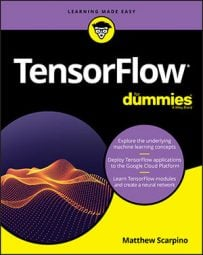##### TensorFlow For DummiesMachine learning applications are fundamentally mathematical, and TensorFlow provides a wealth of routines for performing mathematical operations on tensors. Each routine is represented by a function of the `tf `package, and each function returns a tensor. When it comes to TensorFlow operations, its best to start simple. The following table lists 12 functions that perform basic math operations.

Basic Math Operations

 Function Description `add(x, y, name=None)` Adds two tensors `subtract(x, y, name=None)` Subtracts two tensors `multiply(x, y, name=None)` Multiplies two tensors `divide(x, y, name=None)` Divides the elements of two tensors `div(x, y, name=None)` Divides the elements of two tensors `add_n(inputs, name=None)` Adds multiple tensors `scalar_mul(scalar, x)` Scales a tensor by a scalar value `mod(x, y, name=None)` Performs the modulo operation `abs(x, name=None)` Computes the absolute value `negative(x, name=None)` Negates the tensor's elements `sign(x, name=None)` Extracts the signs of the tensor’s element `reciprocal(x, name=None)` Computes the reciprocals
The first four functions perform element-wise arithmetic. The following code demonstrates how they work:
`a = tf.constant([3., 3., 3.])b = tf.constant([2., 2., 2.])sum = tf.add(a, b)                 # [ 5. 5. 5. ]diff = tf.subtract(a, b)           # [ 1. 1. 1. ]prod = tf.multiply(a, b)           # [ 6. 6. 6. ]quot = tf.divide(a, b)             # [ 1.5 1.5 1.5 ]`
Applications can perform identical operations by using regular Python operators, such as +, -, *, /, and //. For example, the following two lines of code create the same tensor:
`total = tf.add(a, b)               # [ 5. 5. 5. ]total2 = a + b                     # [ 5. 5. 5. ]`
When operating on floating-point values, `div `and `divide `produce the same result. But for integer division, `divide `returns a floating-point result, and `div `returns an integer result. The following code demonstrates the difference between them:
`a = tf.constant([3, 3, 3])b = tf.constant([2, 2, 2])div1 = tf.divide(a, b)             # [ 1.5 1.5 1.5 ]div2 = a / b                       # [ 1.5 1.5 1.5 ]div3 = tf.div(a, b)                # [ 1 1 1 ]div4 = a // b                      # [ 1 1 1 ]`
The `div `function and the `/` operator both perform element-wise division. In contrast, the `divide `function performs Python-style division.